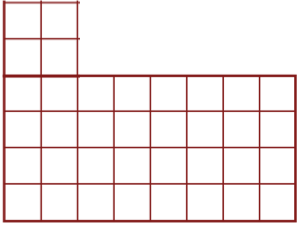# 2 Facts About Teaching AreaJust count the squares!

Are you teaching your class to find the area of shapes?  There are various ways to teach it, but an effective way to teach area is to use what they already know:  arrays and the distributive property.  Since multiplication is such a major concept to teach in third grade, students know arrays backwards and forwards (hehe…yeah that’s the Commutative Property of Multiplication for you!).  They also know how to use the Distributive Property of Multiplication to solve larger arrays.

So, if the students know these concepts, teaching area as Length x Width (does that bring back memories??) is a snap!  I had my students use foam tiles that came with the Go Math! program.  Initially, students were taught to just count the square tiles (gotta hammer it in to them that we are measuring SQUARE UNITS, not just units).Use the formula, L x W

I asked them if they could figure out a faster way to find the area.  Immediately, most said to multiply and made the connection to using an array.  Bingo!  From there, it was full speed ahead with just using the formula (L x W = A).

But what happens when the shapes are irregular shapes?  That’s when the Distributive Property of Multiplication (DPM) takes over the work!  Now, I had to make sure that the students actually remembered how to use the DPM. So I brought out my newest center:  The Distributive Property of Multiplication Ninja Center!

Rather than have just one student at a time use the center, I had the students arrange themselves in groups of 5.  Then I gave each group a part of the center.  The center actually has 20 mats grouped 4 at a time by the factors 5, 6, 7, 8, and 9.  One student was in control of the Answer Key and was designated the Sensei who made sure each Ninja correctly split an array and showed the correct DPM sentence.  This took about 10 minutes which was all I needed  to make sure the students knew exactly how to use he DPM.

From there, it was not hard to teach them to use the DPM to find the area of irregular shaped rectangles.  The students were very savvy on how to bisect the figures to get smaller shapes.  Then they wrote multiplication facts for each array and put them together as a DMP sentence.   We will see how well they do with these kinds of problems as the chapter test is coming up, not to mention the Math portion of the SBAC in May!Use the Distributive Property of Multiplication to find the area of this irregular shape.

What interesting and fun ways do you have to teach area?  Share your ideas here!

Updated 4/27/15:  Check out my newest resource for Area and Perimeter:  The Area and Perimeter Game